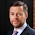## Monday, March 4, 2013

### Fun Calculus AP Activities

Let's face it - calculus is a difficult class.  Class is often the same - go over the homework, lecture on something new, do your homework.  Everyone needs a fun activity now and again - even the best math students appreciate a puzzle, a game, or a new approach.

Here are some of my favorite activities I have found for Calculus on Teachers Pay Teachers:

1)  Derivatives and Integrals Matching Mania - In this activity, students work together to find the integral and first and second derivative of a function.  They then fill in a worksheet to turn in to the teacher.  The equations involve trig functions, log functions, and exponential functions.

2)  Coyote's Instantaneous Velocity - I think this is a great idea to show students what instantaneous velocity means while relating it something that every kid has seen - the coyote falling off the cliff!

3)  Integration by Substitution - A fun activity to have students work on using u-substitution.  Best of all - it's FREE!

4)  Antiderivative Scavenger Hunt - Students will work on finding the antiderivative of functions.  They begin with one function and find its antiderivative somewhere in the room.  They continue until they have found all of the antiderivatives.  A fun way to get the students out of their seats!

5)  Find My Match Graphing Activity - This is my own activity that I was originally going to use for Valentine's Day, but didn't get around to it.  However, I decided that I might like to do it at any time of year, so I didn't use any Valentine Graphics.  Here is the premise...each student is given either an equation, graph of the function, or graph of the derivative of a function.  After several minutes of working with a worksheet to help students organize their thoughts, they walk around the room trying to find their match.  Each group will end up with three students - one with an equation, one with the graph of an original function, and one with a graph of the derivative of the function.

#### 1 comment:

1.Great ideas! I will be sure to use some of them with my tutoring students--all of them do pretty boring in-class activities, hence the need for outside instruction. Thanks for sharing!